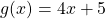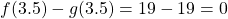# Polynomial Equations

Finding solutions of polynomial equations where the degree of the resulting polynomial is higher than 2 is best done using graphing or CAS technology. However, if there is reason to believe that the solutions are integers, (or the resulting equation is a quadratic) the factor theorem may be used.

# Example

Find all values ofsuch thatGraphing two functions together, letandas shown below.We see that when, both curves have the samevalue.This is also true when:Therefore the expressionsandhave equal value whenor when.

To solve algebraically, we reduce once side to zero by subtracting the terms of one side from both sides:Another way to understand this algebra graphically is to graph the related function, that is,Notice that the-intercepts of the graphhave the samevalues as the intersections of the graphsand. That is because when we subtract two equal values, the answer is zero.

Because;. In the same way,.

# Conclusion

To solve an equation, we may

• Draw the graphs of both and locate the intersections of the graphs. We are looking for thevalues of the intersections.
• Draw the graphand locate the roots of the graphs.

For these two methods technology speeds up the process considerably. Here’s a how to video for this calculation on GeoGebra. Most graphing software has a tool or command for finding intersections and for finding-intercepts.

• To solve the equationalgebraically, we first rearrange to, then solve by factoring if possible.

# Practice

The equations in this applet all have at least one integer solution. If the resulting polynomial has a degree greater than 2, the factor theorem should be used to find the first solution.